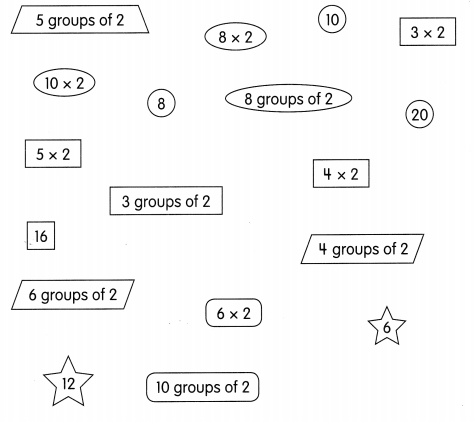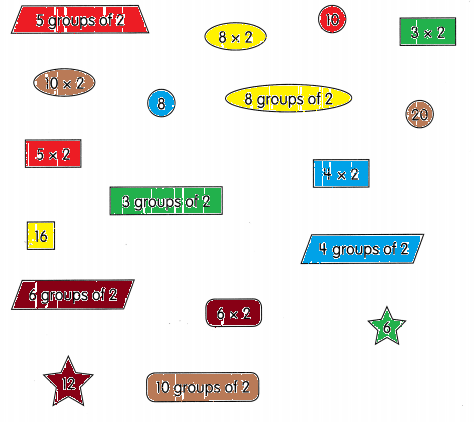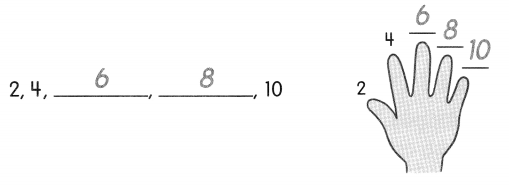# Math in Focus Grade 2 Chapter 6 Practice 1 Answer Key Multiplying 2: Skip-counting

Go through the Math in Focus Grade 2 Workbook Answer Key Chapter 6 Practice 1 Multiplying 2: Skip-counting to finish your assignments.

## Math in Focus Grade 2 Chapter 6 Practice 1 Answer Key Multiplying 2: Skip-counting

Color the shapes.
Use the same color for the shapes that have the same value.

Question 1.Count by 2s.

Then fill in the blanks.

ExampleQuestion 2.
6, 8, 10, ___, ____, ____, 18
6,8,10,12,14,16,18

Question 3.
8, ___, 12, 14, ____, ____ 20
8,10,12,14,16,18,20

Question 4.
___10, 12, 14, ___, ____
8,10,12,14,16,18

Fill in the blanks.

Question 5.
6 × 2 = ____
Explanation:
Multiply the value two with six then we get
6 × 2 = 12

Question 6.
3 × 2 = ___
Explanation:
Multiply the value two with three then we get
3 × 2 = 6

Question 7.
7 × 2 = ____
Explanation:
Multiply the value two with seven then we get
7 × 2 = 14

Question 8.
4 × 2 = ___
Explanation:
Multiply the value two with four then we get
4 × 2 = 8

Question 9.
9 × 2 = ___
Explanation:
Multiply the value two with nine then we get
9 × 2 = 18

Question 10.
5 × 2 = ___
Explanation:
Multiply the value two with five then we get
5 × 2 = 10

Question 11.
8 × 2 = ___
Explanation:
Multiply the value two with eight then we get
8 × 2 = 16

Question 12.
1 × 2 = ___
Explanation:
Multiply the value two with one then we get
1 × 2 = 2

Question 13.
10 × 2 = ___